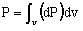# Post-processing quantities (3D)

## Introduction

The post-processing quantities are of two types:

• local quantities, analyzed on all points of the study domain
• global quantities, resulting from an integration, analyzed over the entire study domain or on a part of this domain

## Local quantities

Local quantities available in 3D problems are presented in the table below.

Quantity PyFlux Unit Explanation
Scalar electric potential: V Ve V
Electric field strength: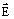E V/m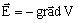Current density: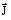J A/m2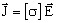Normal component of the current density: Jn Jn A/m2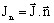Magnetic flux density due to the currents: BJ Bj T (Biot and Savart)
Conductivity: σ Sigma S
Resistivity: ρ Rho Ω.m
Volume density of losses by Joule effect: dP dLossV W/m3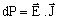## Global quantities

Global quantities available in 3D problems are presented in the table below.

Global quantity Name Unit Explanation
Laplace force on a conductor: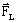Laplace Force N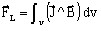Losses by Joule effect: P Losses W